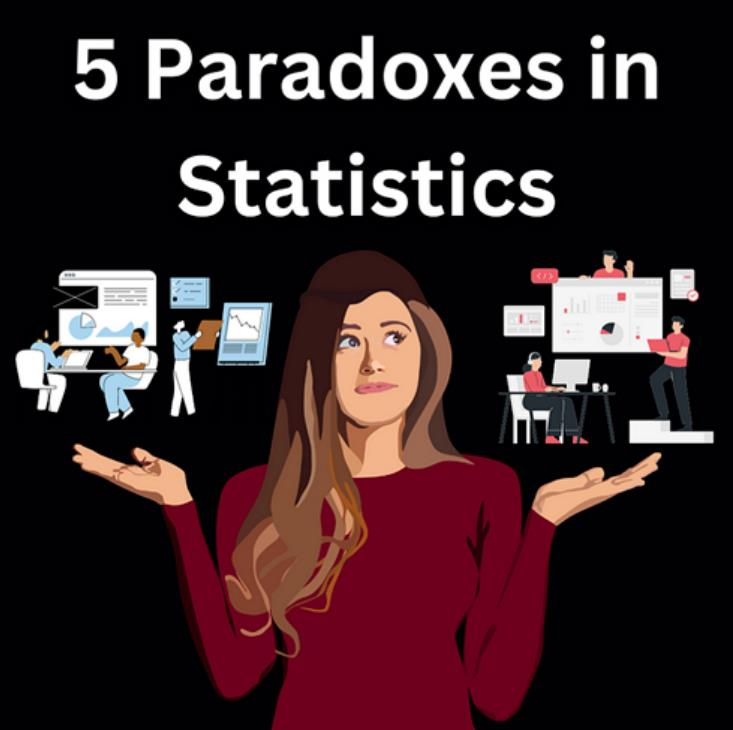## 数据科学家须知：统计学中的5个悖论

14场Kaggle比赛，开启你的数据科学之旅

3. 赌徒谬论（Gambler’s Fallacy）
6. 结论

``````import numpy as np
from sklearn.metrics import accuracy_score

# create imbalanced dataset
y_true = np.array( * 900 +  * 100)
y_pred = np.zeros(1000)

# calculate accuracy
accuracy = accuracy_score(y_true, y_pred)
print('Accuracy:', accuracy)``````

``````import pandas as pd
import numpy as np

# Define variables
normal_count = 9999
fraud_count = 1
false_positives = 499.95
false_negatives = 0

# Calculate precision
precision = fraud_count / (fraud_count + false_positives)
print(f"Precision: {precision:.2f}")

# Calculate recall
recall = fraud_count / (fraud_count + false_negatives)
print(f"Recall: {recall:.2f}")

# Calculate accuracy
true_negatives = normal_count - false_positives
accuracy = (true_negatives + fraud_count) / (normal_count + fraud_count)
print(f"Accuracy: {accuracy:.2f}")

Output:
Precision: 0.00
Recall: 1.00
Accuracy: 0.95``````

``````from sklearn.metrics import confusion_matrix
from sklearn.datasets import make_classification
from sklearn.model_selection import train_test_split
from sklearn.linear_model import LogisticRegression

# generate a binary classification dataset
X, y = make_classification(n_samples=1000, n_features=10, n_informative=5, n_redundant=5, random_state=42)

# split the dataset into train and test sets
X_train, X_test, y_train, y_test = train_test_split(X, y, test_size=0.3, random_state=42)

# train a logistic regression model
model = LogisticRegression(random_state=42)
model.fit(X_train, y_train)

# predict on test set and get the confusion matrix
y_pred = model.predict(X_test)
tn, fp, fn, tp = confusion_matrix(y_test, y_pred).ravel()

# calculate the accuracy, precision, and recall
accuracy = (tp + tn) / (tp + tn + fp + fn)
precision = tp / (tp + fp)
recall = tp / (tp + fn)

print(f"Accuracy: {accuracy:.2f}")
print(f"Precision: {precision:.2f}")
print(f"Recall: {recall:.2f}")

Output:
Accuracy: 0.79
Precision: 0.82
Recall: 0.75``````

3 赌徒谬论（Gambler’s Fallacy）

``````import numpy as np

# Simulate flipping a coin 10 times
results = np.random.randint(0, 2, size=10)
print(f"Coin flips: {results}")

# Count the number of consecutive heads or tails
consecutive = 0
for i in range(1, len(results)):
if results[i] == results[i-1]:
consecutive += 1
else:
consecutive = 0

# Print the result
if consecutive > 0:
print(f"Number of consecutive flips: {consecutive + 1}")
else:
print("No consecutive flips")

Output:
Coin flips: [0 1 0 0 0 0 0 0 1 0]
No consecutive flips``````

``````import pandas as pd

# Create a dataframe
df = pd.DataFrame({'Department': ['A', 'A', 'B', 'B'],
'Gender': ['Male', 'Female', 'Male', 'Female'],
'Applicants': [100, 80, 500, 400],

# Display the dataframe
print(df)

overall_rate = df['Admitted'].sum() / df['Applicants'].sum() * 100

# Calculate admission rates by department and gender
print(department_rates)

Ouput:
0          A    Male         100        60            60.0
1          A  Female          80        40            50.0
2          B    Male         500        40             8.0
3          B  Female         400        70            17.5
Department  Gender
A           Female    50.0
Male      60.0
B           Female    17.5
Male       8.0

``````import pandas as pd
import seaborn as sns

correlation = iris['sepal_length'].corr(iris['sepal_width'])
print('Correlation between sepal length and width:', correlation)

Correlation between sepal length and width: -0.11756978413300208``````

``````setosa = iris[iris['species'] == 'setosa']
correlation_setosa = setosa['sepal_length'].corr(setosa['sepal_width'])
print('Correlation between sepal length and width for setosa:', correlation_setosa)

Correlation between sepal length and width for setosa: 0.7425466856651597``````

1. 准确性悖论告诉我们，仅有准确性不足以评估分类任务，精确度和召回率提供的信息更多。
2. 假阳性悖论强调了理解假阳性成本的重要性。
3. 赌徒谬论提醒我们，每个事件都是独立的，过去的结果不会影响未来的结果。
4. 辛普森悖论表明，汇总数据可以模糊变量之间的关系，并导致错误的结论。
5. 最后，伯克森悖论表明，当从总体中选择非随机样本时，抽样偏差是如何发生的。